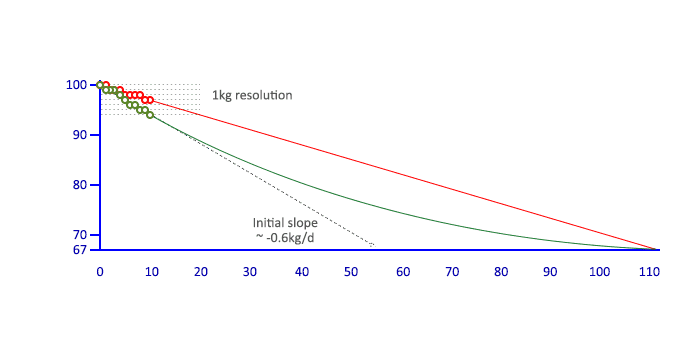# How to create a parabolic formula - For Weight Loss

• B
tomtomtom1
TL;DR Summary
How to create a parabolic formula
Hello all

I was hoping someone can help with the following problem.

I am trying to create a parabolic formula to give me target weight.

For example:-

Day 1 = Target Weight 100kg
Day 112 = Target Weight 67kg

Each day is consecutive - can anyone help me to create a parabolic formula that goes from 100kg in Day 1 down to 67kg in Day 112.

A linear equation is easier but doesn't do it for me a parabolic formula would

Can anyone help?

Thank you.

Mentor
Do you maybe mean exponential instead of parabolic? It makes a difference what you want to base the non-linear term in your equation on (just time or the previous day's weight)...

Gold Member
A couple of cautionary words - in case this is an actual weight loss plan:

1. That's an extremely aggressive timetable. 72 lb. in 112 days - or two pounds every three days. One might call it dangerous. Medical opinions vary, but a good ballpark figure for weight loss is more like 1-2 pounds (.5kg-1kg) per week.

2. If you follow through, you have a date with a hospital for dehydration and electrolyte imbalance (which leads to a heart attack).

3. There is no point in weighing yourself more often than once per week. Your normal weight will vary by several pounds per day - trying to track it any more frequently than weekly will give false readings of your true weight loss.

Last edited:
••zoki85, mfb, phinds and 1 other person
Mentor
Summary:: How to create a parabolic formula

I was hoping someone can help with the following problem.

I am trying to create a parabolic formula to give me target weight.
@tomtomtom1 (if that is your REAL name...) -- Is this more homework?

Mentor
From the mathematical side, a parabola has three degrees of freedom, you only fixed two. There is e.g. a parabola that has the given start and end numbers but goes through 1000 kg in between. There is also a parabola that goes through -100 kg in between. Clearly these examples are nonsense, but there is still a large range of non-nonsense parabolas and no rule given to distinguish between them.

I also don't see what a parabola would do better than a linear function. Keeping the same weight loss per week is easier and probably better for the body, too. And keep the advice of post 3 in mind.

tomtomtom1
From the mathematical side, a parabola has three degrees of freedom, you only fixed two. There is e.g. a parabola that has the given start and end numbers but goes through 1000 kg in between. There is also a parabola that goes through -100 kg in between. Clearly these examples are nonsense, but there is still a large range of non-nonsense parabolas and no rule given to distinguish between them.

I also don't see what a parabola would do better than a linear function. Keeping the same weight loss per week is easier and probably better for the body, too. And keep the advice of post 3 in mind.

Hi mfb

Would some form of logarithmic function work?

Mentor
tomtomtom1
Do you maybe mean exponential instead of parabolic? It makes a difference what you want to base the non-linear term in your equation on (just time or the previous day's weight)...

Berkeman

If an exponential/log function would meet Day 1 = 100kg and Day 112 = 67kg then i would be happy to try that but i just don't know how to generate the function that meets those requirements.

Mentor
@tomtomtom1 (if that is your REAL name...) -- Is this more homework?
You didn't respond to @berkeman's question.

An exponential function (decaying) would look like this: ##W(x) = W_0e^{-kx}##, where k is a positive constant. Using your given information, W(1) = 100 and W(112) = 67, I get an exponential function that is close to being a straight line.

Homework Helper
If an exponential/log function would meet Day 1 = 100kg and Day 112 = 67kg then i would be happy to try that but i just don't know how to generate the function that meets those requirements.
Pick any function whatsoever. If it does not happen to hit the points where W(1)=100 and W(112)=67 then add a linear function to make up the difference.

This would be a [trivial] example of Lagrange interpolation.

A different approach is the one that @Mark44 used. Find a function that has two or more tunable parameters and tune them so that W(1) = 100 and W(112) = 67.

Gold Member
I think jedish's question really needs an answer: is this homework?

Because if it isn't, one must ask how much use it can be. If the OP is
- measuring OP's weight daily,
- and that can vary by as much as a kg,
- and OP's scale only measures to a precision of one kg
- and OP is trying to track a curve that varies by less than one kg per day...Also notice that - to hit the target weight by the target date and maintain a slope that levels off the farther one goes - the initial slope must be steeper than average i.e. the OP will have to lose a greater than average weight in the first few weeks.

Essentially the OP has to lose something on the order of 1 kg every two days for the first couple of weeks.

Good luck doing that without suffering dehydration - or worse.

Last edited: